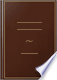Søk Bilder Maps Play YouTube Nyheter Gmail Disk Mer »
Logg på
 Bøker Bok 21–30 av 192 på All the interior angles of any rectilineal figure, together with four right angles,...All the interior angles of any rectilineal figure, together with four right angles, are equal to twice as many right angles as the figure has sides.Elements of Geometry: Containing the First Six Books of Euclid : with a ... - Side 308
av John Playfair - 1832 - 333 sider
Uten tilgangsbegrensning - Om denne bokenThe Elements of Euclid: Viz. the First Six Books, Together with the Eleventh ...

Euclides - 1816 - 528 sider
...&c. QED COR. 1. All the interior angles of any rectilineal figure, together with four right angles, are equal to twice as many right angles as the -figure has sides. For any rectilineal figure ABCDE, can be divided into as many triangles as the figure has sides, by...
Uten tilgangsbegrensning - Om denne bokenEuclid's Elements of Geometry: The Six First Books. To which are Added ...

Rev. John Allen - 1822 - 494 sider
...as many right angles, except four, as the figure has sides ; and all tbe angles of these triangles are equal to twice as many right angles as the figure has sides (32. 1 /CM.), therefore the angles of these triangles which are at their common vertex A, being those...
Uten tilgangsbegrensning - Om denne bokenThe First Six Books with Notes

Euclid - 1822 - 179 sider
...equal (i)Prop. 13. to two right angles (1), therefore all the external angles, with all the internal, are equal to twice as many right angles as the figure has sides ; but the internal angles, together with four right angles, are equal to twice as many rightangles...
Uten tilgangsbegrensning - Om denne bokenEuclid's Elements of Geometry: The Six First Books. To which are Added ...

Rev. John Allen - 1822 - 494 sider
...two right angles [13. 1} ; therefore all the exterior and interior angles of the figure are together equal to twice as many right angles as the figure has sides ; but the interior angles are equal to twice as many right angles, except four, as the figure has sides...
Uten tilgangsbegrensning - Om denne bokenA Course of Mathematics: For the Use of Academies, as Well as Private ...

Charles Hutton - 1822
...triangles, is equal to two right angles (th. 17) ; therefore the sum of the angles of all the triangles is equal to twice as many right angles as the figure has sides. But the sum of all the angles about the point p, which are so / many of the angles of the triangles,...
Uten tilgangsbegrensning - Om denne bokenA treatise on navigation, and nautical astronomy

Edward Riddle - 1824
...angles as the figure has sides. But all the interior angles, and four right angles, are also together equal to twice as many right angles as the figure has sides, (Theo. 25.) Hence the interior and the exterior angles of the figure are, together, equal to the interior...
Uten tilgangsbegrensning - Om denne bokenA Popular Course of Pure and Mixed Mathematics ...: With Tables of ...

Peter Nicholson - 1825 - 372 sider
...QE I). Cor. 1 . All the interior angles of any rectilínea] figure, together with four right angles, are equal to twice as many right angles as the figure has sides. For any rectilineal figure ABCDE can be divided into as many triangles as the figure has sides, by...
Uten tilgangsbegrensning - Om denne bokenElements of arithmetic, algebra and geometry

George Lees - 1826 - 207 sider
...are also together equal to two right angles. Cor. All the interior angles of any rectilineal figure are equal to twice as many right angles as the figure has sides, wanting four right angles. For any rectilineal figure, ABCDE can be divided into as many triangles as the figure...
Uten tilgangsbegrensning - Om denne bokenThe Elements of Euclid: The Errors by which Theon, Or Others, Have Long ...

Robert Simson - 1827 - 513 sider
...E, zi. COR. 1. All the interior angles of any rectilineal figure, together with four right angles, are equal to twice as many right angles as the figure has sides. For any rectilineal figure ABCDE, can be divided into as many triangles as the figure has sides, by...
Uten tilgangsbegrensning - Om denne bokenElements of Geometry: With Notes

John Radford Young - 1827 - 208 sider
...in each triangle amounts to two right angles, therefore the angles of all the triangles are together equal to twice as many right angles as the figure has sides, that is to say, the sum of the angles of the polygon, together with those about the point within it,...
Uten tilgangsbegrensning - Om denne boken